# Math Clip Art--Clock Art--Clock Face Showing 5

## Looking for Clock Clip Art?

This is part of a series of math clip art images that show the time in 5-minute increments. This entire collection covers each of the hours from 12 to 11. Use these clip art images with PowerPoint, GoogleSlides, and Media4Math's proprietary Slide Show Creator.

### To see the complete collection of clock clip art, click on this link. This includes animated clip art and digital clock faces.

The following section includes background information on telling time. This includes a video that gives the basics of telling time. Then there is a review of telling time. Use these resources to supplement your lessons on learning to tell time.

## Video: Telling Time

To review telling time, watch this video. (The transcript is also included.)

## Telling Time

This video goes through the basics of telling time by showing how to read the hour and minute hands. Use this video in your unit of instruction for telling t...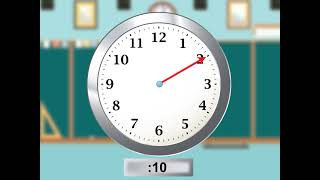The video was uploaded on 9/6/2022.

You can view the video here.

The video lasts for 2 minutes and 12 seconds.

Video Transcript

When you look at a clock, you can read the hour and the minutes. >Here is a blank clock face. Let's start by looking at the hour hand. It is the shorter of the two clock hands and looks like this.

The hour hand varies in value from 1 to 12. Here is a tour of times around the clock using the hour hand.

12 o'clock.

1 o'clock.

2 o'clock.

3 o'clock.

4 o'clock.

5 o'clock.

6 o'clock.

7 o'clock.

8 o'clock.

9 o'clock.

10 o'clock.

11 o'clock.

And back to 12 o'clock.

The minute hand is the longer of the two clock hands. This is what it looks like. The minute hand varies in value from 0 to 60.

Here is a tour of times around the clock in 5-minute increments.

5 minutes after the hour.

10 minutes after the hour.

15 minutes after the hour.

20 minutes after the hour.

25 minutes after the hour.

30 minutes after the hour.

35 minutes after the hour.

40 minutes after the hour.

45 minutes after the hour.

50 minutes after the hour.

55 minutes after the hour.

0 minutes after the hour.

Telling time involves reading both the hour and minute hand. Here are some examples. This is 12 o'clock. Both the hour and minute hands point to 12. This is 12:15. The hour hand is a bit past 12 and the minute hand points to 3.

This is 12:30. The hour hand is between 12 and 1, and the minute hand points to 6.

This is 12:45. The hour hand is closer to 1 and the minute hand points to 9.

This is 1 o'clock. The hour hand points to 1 and the minute hand points to 12.

This pattern repeats for every hour and minute.

## The Hour Hand

When you look at a clock, you can read the hour and the minutes. Here is a blank clock face. Look at the arrangement of numbers on the clock face. Make a note of the following items:

• The numbers go from 1 to 12.
• There are additional tic marks between the main numbers.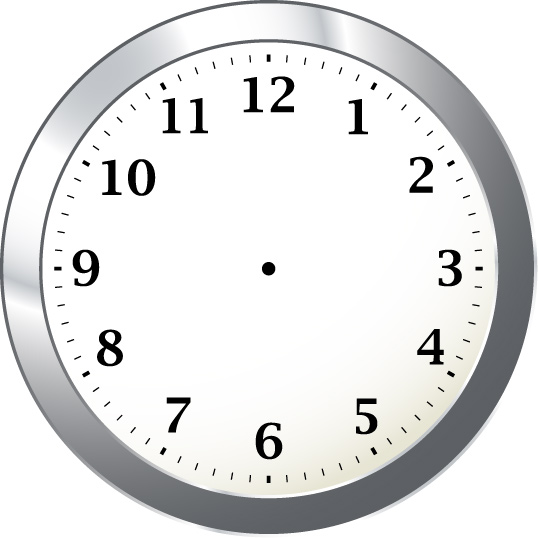Let’s start by looking at the hour hand. It is the shorter of the two clock hands and looks like this.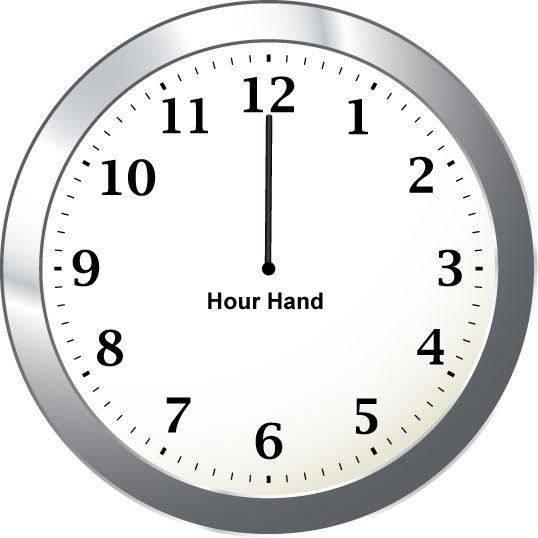The hour hand varies in value from 1 to 12. Here are some sample times using the hour hand.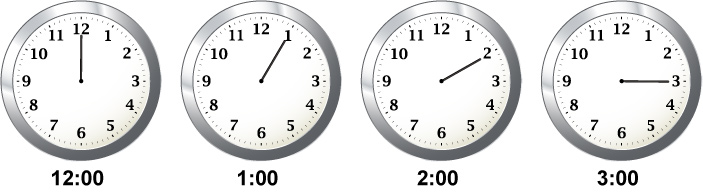## The Minute Hand

The minute hand is the longer of the two clock hands. This is what it looks like.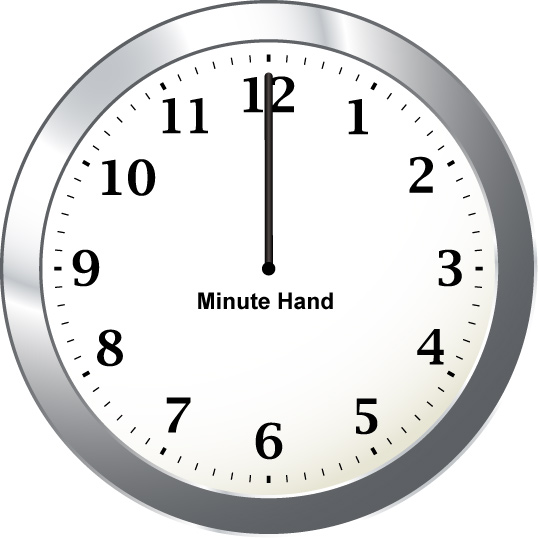The minute hand varies in value from 0 to 60. Here are some sample times using the minute hand.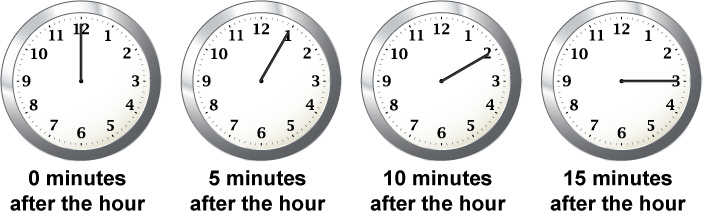## Why Are Hours Measured From 1-12 Not 1-10?

We are so accustomed to counting objects from 1-10 and in multiples of 10. Why are minutes and hours different? Why are minutes counted from 1 to 60? Why are hours counted from 1 to 12?

When we count from 1 to 10, we are using the base 10 system. The numbers cycle through groups of 10. But with clocks there is a different number system at work. Can you tell what the base is?

Clocks rely on a base 60 system. Think of the minute hand. After 60 minutes, the clock advances one hour and the minutes start again. This is an example of counting in base 60.

Why 60? With time measurement, we want to keep precise track of the time. The number 60 has many more factors, so we can measure time in fractions of 60 that are numbers that can be easily be remembered. Using base 10 with clocks would result in awkward fractions of time.

This table shows the fractions of an hour that result in whole number minutes of time that are easy to remember.

 Minutes Fraction of an Hour 1 1/60 2 1/30 5 1/12 6 1/10 10 1/6 12 1/5 15 1/4 20 1/3 30 1/2

With base 60, there are so many more fractional amounts of time that are whole numbers, and they're easy to remember. Try this with base 10 and you won't end up with as many, easy-to-remember fractions.